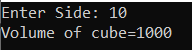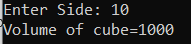# Volume of Cube in C#

## Volume of Cube Program in C# with Examples

In this article, I am going to discuss how to calculate the Volume of Cube in C# with Examples. Please read our previous article, where we discussed How to Calculate the Area of Cube in C#. Here, in this article, first, we will discuss what is Cube, then we will discuss how to calculate the Volume of a Cube and finally, we will see how to implement the volume of cube program in C#.

##### What is a Cube?

A cube is a 3D (Three Dimensional) shape. It has six faces, 8 vertices, and 12 edges and all the six faces of a cube are squares.

##### How to Calculate the Volume of a Cube?

The volume of an object is defined as the amount of space that a solid occupies
Volume of cube (V)= a*a*a = a3
Where a is the side of the cube
V is the volume of the cube

Example:
Input: side, a = 10
Output:
Volume = a3
= 103
= 1000

In this program, we are going to use the following Data types, Function

1. int: Integer variables are variables that must take an integer value (0, 1, 2, …). A special kind of integer variable is binary variables. Example: int a= 10
2. float: Floating-point numbers are used for decimal and exponential values. It is a primitive data type. It has 6 decimal digits of precision. Example: float b = 6.3
3. Pow(): The function pow() is used to calculate the power raised to the base value. It takes two arguments. Example: the syntax of pow() is double pow(double val1, double val2);
##### Logic to Calculate the Volume of Cube in C#

To find the volume of a cube, we need to follow the below steps

1. First, we will define a variable, side, volume.
2. Then we will assign the value of side(side). Example side=10;
3. After that, we will calculate the volume of the cube by formula. side*side*side
4. At last, the result will store in the volume variable and show the output.
##### Example: Volume of Cube Program in C#

The following C# Program will calculate the volume of a cube.

```using System;
public class VolumeOfCube
{
public static void Main()
{
Console.Write("Enter Side: ");
decimal Volume = Side * Side * Side;
Console.WriteLine("Volume of cube=" + Volume);
}
}
```
###### Output:##### Example: Volume of Cube Program in C# using Pow function
```using System;
public class VolumeOfCube
{
public static void Main()
{
Console.Write("Enter Side: ");